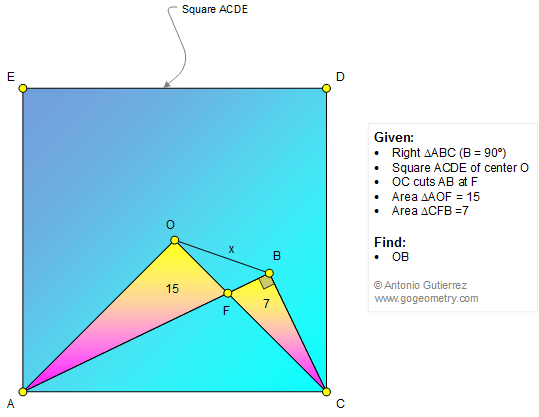#Problem 1310: Square, Center, Right Triangle, Area, Measurement. Teaching, Tutoring.

 < PREVIOUS PROBLEM  |  NEXT PROBLEM > The figure below shows a right triangle ABC (angle B = 90 degrees) and a square ACDE of center O so that OC cuts AB at F. If the areas of the triangles AOF and CFB are 15 and 7, respectively, find the measure of OB.Home | Geometry | Search | Problems | All Problems | Open Problems | Visual Index | 1301-1310 | Triangle | Square | Triangle Area | View or Post a solution | by Antonio Gutierrez